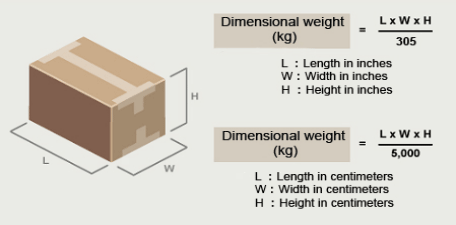##### Volumetric weightVolumetric weight refers to the overall size of a parcel and is measured in volumetric kilograms. Volumetric weight can be calculated by multiplying the length, width and height of a parcel (in cm) and dividing that figure by 5000 (some carriers use a divisor of 4000).

You may often find that the price of your shipment is dictated by the volumetric weight of your parcel(s) rather than the physical weight. This is because our pricing is calculated based on whichever is the greater out of the volumetric weight and the physical weight.

For example, you could have a box of feathers that is quite large, say 100 cm X 50 cm X 50 cm but is relatively lightweight at 5kgs. Using the above calculation (length X width X height / 5000), the volume of this parcel is 50 volumetric kilograms. As the volume 'outweighs' the physical weight of 5kgs, the price is based on 50 kilograms. For this reason it is extremely important to measure parcel(s) their widest, longest and highest points. Any bulges, handles, tags or packaging that could break the beam of a measuring laser must be included.

It's worth noting that it works the other way around too. You could have a small box of heavy metal components (30 cm x 30 cm x 20cm) weighing 10kg in physical weight. The volume of this parcel is 5.4kgs. So in this instance the volumetric weight is lower than the physical weight meaning that the price would be calculated on 10kg.Foreign Parcel Service D-light international is a leading international courier service provider that has been serving the needs of its clients since its establishment.